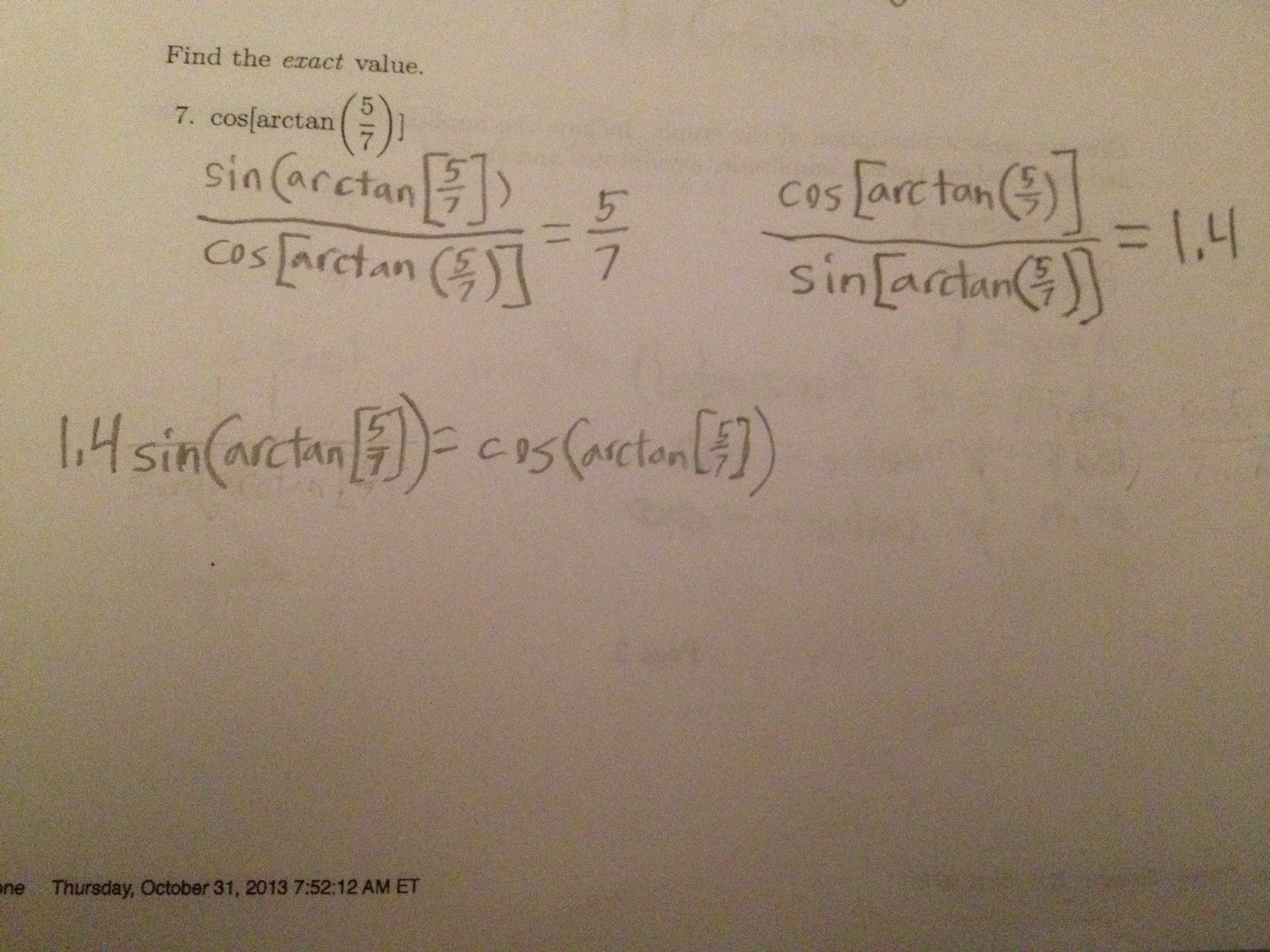Categories

# cos[arctan(5/7)]…What’s up with this cool work?

via Tina

## 4 replies on “cos[arctan(5/7)]…”

So we start with tan (arctan (5/7)), then we’ve got cot(arctan(5/7)) = 7/5 That’s reassuring and logical. Then we have cot(arctan(5/7))*sin(arctan(7/5)) = cos(arctan(5/7))
Since cotx = cosx/sinx the student extended that to any input x – in this case the input is the complicated arctan(5/7)

Generally true? Is it even true in this case? I like that the student seems to understand that the input of arctan(5/7) is an angle input.

It’s definitely progress. Now square both sides and you’re almost there!

I like this approach, one good way to start the problem.

I wish that the kid would learn that you should draw a triangle or a unit circle whenever you’re stuck on a trig problem of any sort.

Algebraically, though, barryrsmith’s idea of squaring both sides next is a good way to proceed.

I expected students to solve this by drawing a triangle, but thought it was interesting what he came up with when he forgot that approach!